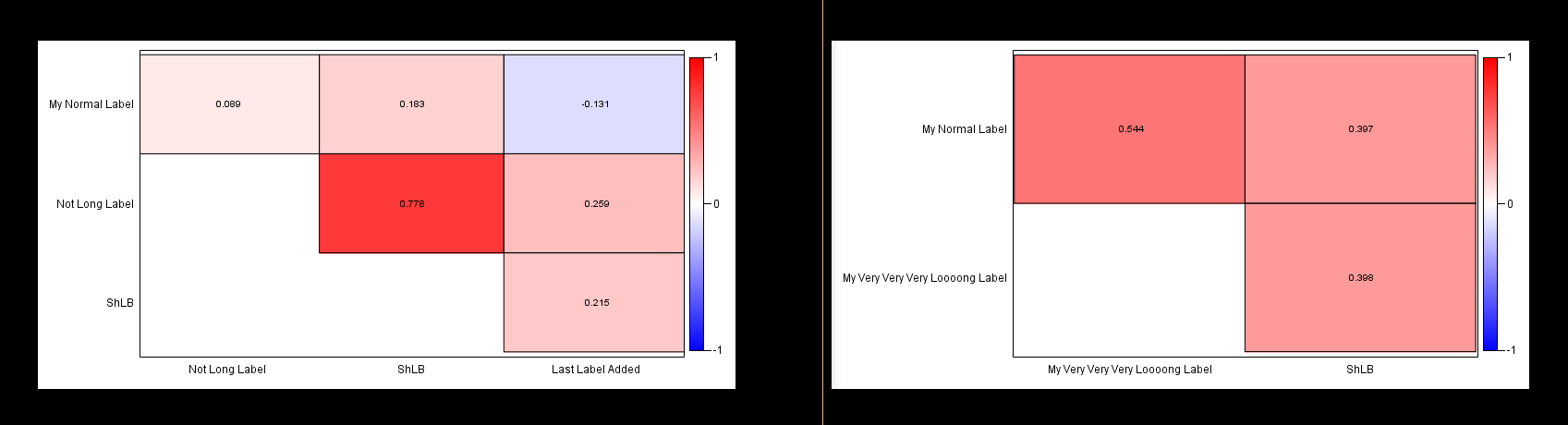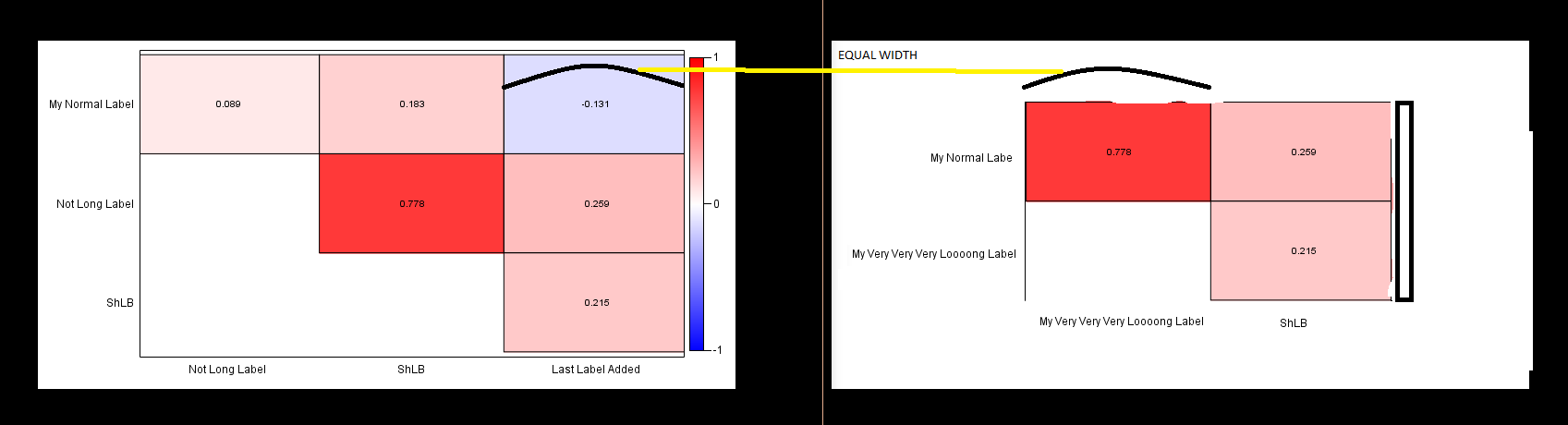## How to keep the same box dimensions in different heatmaps

rHello, I will try to be the most detailed as possible.

Running two different Heatmaps for correlations but with a different number of variables, what SAS is usually doing is to resize the x and y axes to fit all the variables in the same box with prespecified dimension.

Therefore, if I run the same code with 3 or 4 groups each correlation (category) will be fit in a different space, with dimension calculated according the label length and number of categories.But what I would like to have in this case is to resize the box allocated to the graph, allowing white space on the borders of the images (before the labels on the left, after the index of colors on the right and so on..) in order to have the same dimension for each category.

Something like this (created with paint, as you can image):Is this possible?

This is the code to reproduce the graphs printed here above, making use of the sashelp.leutest datasets:

``````/*Creating the 2 correlation datasets for the HeatMap example*/

proc sort data=sashelp.leutest out=leu00a(keep=x1 x2 x3); by x1 x2 x3; run;
proc sort data=sashelp.leutest out=leu00b(keep=x4 x5 x6 x7); by x4 x5 x6 x7; run;

ods output  PearsonCorr=PEA00a(drop=p:);
proc corr data = leu00a pearson nosimple ;
var _NUMERIC_;
run;

ods output  PearsonCorr=PEA00b(drop=P:);
proc corr data = leu00b pearson nosimple ;
var _NUMERIC_;
run;

data PEA01a;
set PEA00a;
length ord1 ord2 \$50 ordn1 ordn2 myval 8.;
array MYX[*] X:;
do k=_n_+1 to dim(MYX);
ordn1=_n_;
ordn2=k;
myval=MYX[k];
if ordn1=1 then ord1='My Normal Label';
if ordn1=2 then ord1='My Very Very Very Loooong Label';
if ordn1=3 then ord1='ShLB';
if ordn2=1 then ord2='My Normal Label';
if ordn2=2 then ord2='My Very Very Very Loooong Label';
if ordn2=3 then ord2='ShLB';
output;
end;

keep ordn1 ordn2 ord1 ord2 myval;
run;
proc sort; by ordn1 ordn2; run;

data PEA01b;
set PEA00b;
length ord1 ord2 \$50 ordn1 ordn2 myval 8.;
array MYX[*] X:;
do k=_n_+1 to dim(MYX);
ordn1=_n_;
ordn2=k;
myval=MYX[k];
if ordn1=1 then ord1='My Normal Label';
if ordn1=2 then ord1='Not Long Label';
if ordn1=3 then ord1='ShLB';
if ordn1=4 then ord1='Last Label Added';
if ordn2=1 then ord2='My Normal Label';
if ordn2=2 then ord2='Not Long Label';
if ordn2=3 then ord2='ShLB';
if ordn2=4 then ord2='Last Label Added';
output;
end;
keep ordn1 ordn2 ord1 ord2 myval;
run;
proc sort; by ordn1 ordn2; run;

/*Template for the HeatMap*/
proc template;
define statgraph MYHEAT;
BeginGraph / designwidth=20 cm designheight=10 cm;
rangeattrmap name='RANGEMAP';
range -1 - 0 / rangecolormodel=(blue white);
range 0 < - 1 / rangecolormodel=(white red);
endrangeattrmap;
rangeattrvar var=MYVAL attrvar=ATRVAR attrmap='RANGEMAP';
layout overlay / yaxisopts=(discreteopts=(tickvaluefitpolicy=none) display=(tickvalues) reverse=true )
xaxisopts=(discreteopts=(tickvaluefitpolicy=rotate) display=(tickvalues));
heatmapparm y=ord1 x=ord2 colorresponse=ATRVAR / name="heatmap";
heatmapparm y=ord1 x=ord2 colorresponse=ATRVAR / display=all includemissingcolor=false fillattrs=(transparency=1);
textplot y=ord1 x=ord2 text=eval(put(myval, 6.3)) ;
continuouslegend "heatmap";
endlayout;
EndGraph;
end;
run;

/*Writing the HeatMaps*/
ods graphics on / reset  border=off imagename="FIRSTIMG" imagefmt=png  ;
ods listing gpath="C:\\MYFOLDER\";
options nodate ;
proc sgrender data=PEA01A template=MYHEAT ;
run;
ods graphics off;

ods graphics on / reset  border=off imagename="SECONDIMG" imagefmt=png  ;
ods listing gpath="C:\\MYFOLDER\";
options nodate ;
proc sgrender data=PEA01B template=MYHEAT ;
run;
ods graphics off;
``````

I would prefer not imputing the calculated space on the sides of the graphs becase this should run on a lot of different numbers of groups. I'm not much interested in the final dimension of the png, just on the plotted square.

Thank you very much for any suggestion.

2 REPLIES 2

## Re: How to keep the same box dimensions in different heatmaps

There is an example here: http://support.sas.com/kb/35/156.html

that is a bit of overkill as it has two different graphs but the one on the left ("Emerging Issues") shows something that with appropriate axis controls may give you a handle on what you want

Or add dummy levels to the data so all of the comparison have the same numbers of groups.

## Re: How to keep the same box dimensions in different heatmaps

I'm not sure if you're pointing me to the option

``pad=(top=5px right=5px)``

Is this correct?

Adding dummy variables would move the color index to the very end of the graph, not beside the border.

Using the option pad I can find some combination, but now, what about the legend area?

I know that I can set the maxlegendarea in the ods graphics statement, but what can I do to set a minimum for the legend area?

Thanks

Discussion stats
• 2 replies
• 718 views
• 0 likes
• 2 in conversation